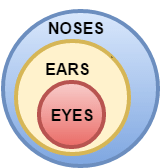# Logical Reasoning for Bank and other Government exams - Set 2

In a certain code language, ‘sea is deep’ means ‘213’, ‘sky is blue’ means ‘514’ and ‘sea looks blue’ means ‘264’

What number is the code for ‘sky’?

a. 1
b. 2
c. 3
d. 4
e. 5
 Answer  Explanation ANSWER: 5 Explanation: In the first and second statements, the common digit is ‘1’ and the common word is ‘is’.So, ‘1’ is the code for ‘is’.In the second and third statements, the common digit is ‘4’ and the common word is ‘blue’.So, ‘4’ is the code for ‘blue’.Thus, in the second statement, ‘5’ is the code for ‘sky’.In the first and third statements, the common digit is ‘2’ and the common word is ‘sea’.So, ‘2’ is the code for ‘sea’.Thus, in the first statement, ‘3’ is the code for ‘deep’.In the third statement, ‘6’ is the code for ‘looks’.

In a certain code language, ‘sea is deep’ means ‘213’, ‘sky is blue’ means ‘514’ and ‘sea looks blue’ means ‘264’

What number is the code for ‘is’?

a. 1
b. 2
c. 3
d. 4
e. 5
 Answer  Explanation ANSWER: 1 Explanation: In the first and second statements, the common digit is ‘1’ and the common word is ‘is’.So, ‘1’ is the code for ‘is’.In the second and third statements, the common digit is ‘4’ and the common word is ‘blue’.So, ‘4’ is the code for ‘blue’.Thus, in the second statement, ‘5’ is the code for ‘sky’.In the first and third statements, the common digit is ‘2’ and the common word is ‘sea’.So, ‘2’ is the code for ‘sea’.Thus, in the first statement, ‘3’ is the code for ‘deep’.In the third statement, ‘6’ is the code for ‘looks’.

3)   There are 64 players in a knock out tournament and every player is ranked (seeded) from 1 - 64.

The matches are played in such a manner that in round one the 1st seeded player plays with the 64th, 2nd with the 63rd and so on.
The players who win move on to the next round whereas others are out of the competition.

In second round, the winner of match 1 will play winner of the last match (which was between seed 32 and seed 33), and winner of match 2 will meet the winner of second last match in round 1 and so forth.

Thus, after all rounds winner is declared.

In a tournament of 128 players, who will play 40 in round 2 if there are no upsets?

a. 25
b. 26
c. 27
d. 28
 Answer  Explanation ANSWER: 25 Explanation: In round 2 there will be 64 players. So, the sum of seeding will be 65.The opponent of 40 will be, 65 - 40 = 25

4)   Choose the odd pair of words.

a. China – Beijing
b. Russia – Moscow
d. India – New Delhi
e. Japan – Ottawa
 Answer  Explanation ANSWER: Japan – Ottawa Explanation: In all other pairs, second term is the capital city of country denoted in the first.

5)   Choose the odd pair.

a. Bottle – Wine
b. Ball – Bat
c. Cup – Tea
d. Pitcher – Water
 Answer  Explanation ANSWER: Ball – Bat Explanation: In all the other pairs, the first is used to hold the second.

1. Rage, Raider, Rattler, Razor, Reaper, Rictor and Rogue are sitting in a circle facing at the centre.
2. Reaper is the neighbour Rage and Razor.
3. Rogue is not between Rictor and Rattler.
4. Rictor is to the immediate right of Rage.

What is the position of Rattler?

a. Third to the left of Reaper
b. To the immediate left of Rage
c. Second to the right of Rictor
d. Immediate left of Raider
 Answer  Explanation ANSWER: Second to the right of Rictor Explanation: Rictor is to immediate right of Rage.Reaper is the neighbor of Rage and Razor i.e. Reaper is between Rage and RazorRogue is not between Rictor and Rattler. So, Raider is between Rictor and Rattler.Thus, the arrangement is as shown –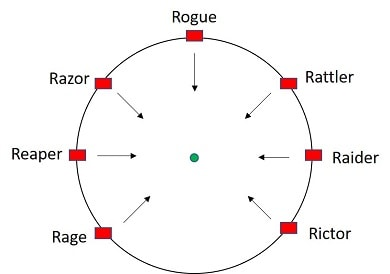Rattler is second to the right of Rictor

1. Rage, Raider, Rattler, Razor, Reaper, Rictor and Rogue are sitting in a circle facing at the centre.
2. Reaper is the neighbour Rage and Razor.
3. Rogue is not between Rictor and Rattler.
4. Rictor is to the immediate right of Rage.

Who are the neighbors of Raider?

a. Rictor and Rattler
b. Rage and Rictor
c. Rattler and Razor
 Answer  Explanation ANSWER: Rictor and Rattler Explanation: Rictor is to immediate right of Rage.Reaper is the neighbor of Rage and Razor i.e. Reaper is between Rage and RazorRogue is not between Rictor and Rattler. So, Raider is between Rictor and Rattler.Thus, the arrangement is as shown –Rictor and Rattler are the neighbors of Raider.

8)   In the following questions, one term in the number series is wrong. Find the wrong term.

2, 3, 5, 8, 13, 20, 34

a. 8
b. 13
c. 20
d. 34
 Answer  Explanation ANSWER: 20 Explanation: The pattern in the given series is -2 + 3 = 55 + 3 = 88 + 5 = 1313 + 8 = 21So, the wrong number is 20.It should be replaced by 21.

9)   In the following question, one term in the number series is wrong. Find the wrong term.

196, 169, 144, 121, 100, 80

a. 144
b. 121
c. 100
d. 80
 Answer  Explanation ANSWER: 80 Explanation: The pattern in the given series is - 142 132 122 112 102 92The wrong number is 80.It should be replaced by 92 = 81

10)   In the question below is given a statement followed by two courses of action numbered I and II. You have to assume everything in the statement to be true and based on the information given in the statement, decide which of the suggested courses of action logically follow(s) for pursuing.

(A) If only I follows
(B) If only II follows
(C) If either I or II follows
(D) If neither I nor II follow
(E) If both I and II follow.

Statement - The migration from villages to cities is detrimental to development of villages.

Courses of Action - I. Sufficient number of colleges should be set up in rural area and a special boost should be provided to the employers to set up relevant industries there.

II. Employment generation scheme should be launched in rural areas.

a. Only I follows
b. Only II follows
c. None follow
d. Neither I nor II follows
e. Both I and II follow
 Answer  Explanation ANSWER: Both I and II follow Explanation: Mass migration occurs due to the lack of opportunities in local area. It can be prevented by making conditions favourable for those residing in villages. Hence, both I and II follow.

11)   In the question below is given a statement followed by two courses of action numbered I and II. You have to assume everything in the statement to be true and based on the information given in the statement, decide which of the suggested courses of action logically follow(s) for pursuing.

(A) If only I follows
(B) If only II follows
(C) If either I or II follows
(D) If neither I nor II follow
(E) If both I and II follow.

Statement - America is not likely to grant Pakistan’s request for \$300 billion fast disbursing loan for the current year.

Courses of Action - I. Pakistan should ask other countries for the loan.

II. Pakistan should talk to America to grant the loan giving it persuasive reasons and explaining the urgency.

a. Only I follows
b. Only II follows
c. Either I or II follows
d. Neither I nor II follow
e. Both I and II follow
 Answer  Explanation ANSWER: Either I or II follows Explanation: Here, there can be two solutions to the problem. i) Pakistan can either stress its urgency and persuade America itself to grant the loanii) It shall explore the opportunity of getting the loan from some other country. Thus, either I or II can follow.

12)   In the following question, a statement/group of statements is given followed by some conclusions. Without resolving anything yourself, choose the conclusion which logically follows from the statement(s).

Statement - Oligopoly is characterised by presence of competition.

The 5G broadband services are provided only by Company X.

a. The X company is a corrupt company.
b. The X company is publicly owned.
c. The X company’s market is monopolistic.
d. The X company has many competitors.
 Answer  Explanation ANSWER: The X company’s market is monopolistic. Explanation: No explanation is available for this question!

13)   In the following question, a statement/group of statements is given followed by some conclusions. Without resolving anything yourself, choose the conclusion which logically follows from the statement(s).

Statement - All that glitters is not gold.

a. Non-metals also glitter.
b. Not all gems glitter.
c. Only diamond glitters.
d. There may be deception in glittering things.
 Answer  Explanation ANSWER: There may be deception in glittering things. Explanation: No explanation is available for this question!

14)   Prajakta moves towards South-East a distance of 70 km, then she moves towards West and travels a distance of 140 km.
From here she moves towards North-West a distance of 70 km and finally she moves a distance of 40 km towards east.

How far is she now from the starting point?

a. 10 km
b. 20 km
c. 30 km
d. 40 km
 Answer  Explanation ANSWER: 10 km Explanation: Required distance = AE = 140 - 40 = 10 km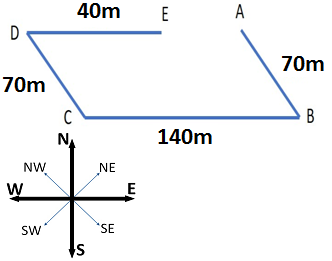15)   If South-East becomes North, North-East becomes West and so on. What will West become?

a. South-East
b. South-West
c. North-East
d. North-West
 Answer  Explanation ANSWER: South-East Explanation: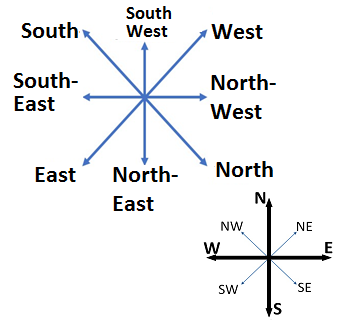1. In a family of six persons, L, M, N, F, P, Q, there are two married couples.
2. F is grandmother of L and mother of M.
3. N is wife of M and mother of Q.
4. Q is the grand-daughter of P.

Who among the following is one of the couples?

a. N-F
b. F-P
c. P-M
d. None of these
 Answer  Explanation ANSWER: F-P Explanation: N is the wife of M.So, one couple is M-N. Now, F is the grandmother of L. M is the son of F and his wife N is the mother of Q.So, F is also grandmother of Q. But Q is the granddaughter of P.So, P is the grandfather of Q and the husband of F.Thus F-P is another couple.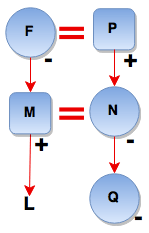1. M \$ N means M is the mother of N
2. M # N means M is the father of N
3. M @ N means M is the husband of N
4. M % N means M is the daughter of N

P  @ Q \$ M # T indicates what relationship of P with T?

a. Paternal grandmother
b. Paternal grandfather
c. Maternal grandfather
d. Maternal grandmother
 Answer  Explanation ANSWER: Paternal grandfather Explanation: P @ Q \$ M # T means P is the husband of Q who is the mother of M who is the father of T i.e. P is the father of T’s father. P is T’s paternal grandfather.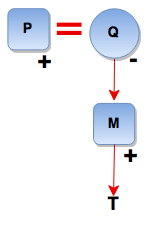18)   How many times do the hands of a clock coincide in a day?

a. 11
b. 22
c. 12
d. 24

Explanation:
The hands of a clock coincide 11 times in every 12 hours (Since between 11 and 1, they coincide only once, i.e., at 12 o'clock).

 AM 12 1.05 2.11 3.16 4.22 5.27 6.33 7.38 8.44 9.49 10.55 PM 12 1.05 2.11 3.16 4.22 5.27 6.33 7.38 8.44 9.49 10.55
The hands overlap about every 65 minutes, not every 60 minutes.
Therefore, The hands coincide 22 times in a day.

19)   In the question below is given a statement followed by two conclusions numbered I and II.

(A) If only I follows
(B) If only II follows
(C) If either I or II follows
(D) If neither I nor II follows
(E) If both I and II follow.

Statement - I. Some cables are keyboard.
II. All cables are monitors.

Conclusion - I. Some monitors are keyboard.
II. Some monitors are not keyboard.

a. Only I follows
b. Only II follows
c. Either I or II follows
d. Neither I nor II follows
e. Both I and II follow
 Answer  Explanation ANSWER: Only I follows Explanation: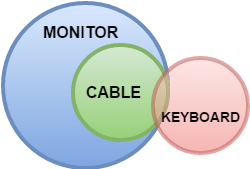20)   In the question below is given a statement followed by two conclusions numbered I and II.

(A) If only I follows
(B) If only II follows
(C) If either I or II follows
(D) If neither I nor II follows
(E) If both I and II follow.

Statement - I. All eyes are ears.
II. All ears are noses.

Conclusion - I. No eyes are ears.
II. Some noses are eyes.

a. Only I follows
b. Only II follows
c. Either I or II follows
d. Neither I nor II follows
e. Both I and II follow
 Answer  Explanation ANSWER: Only II follows Explanation: